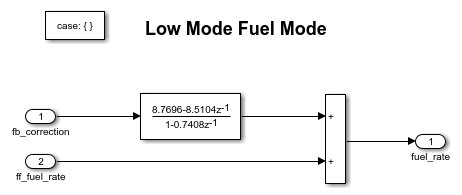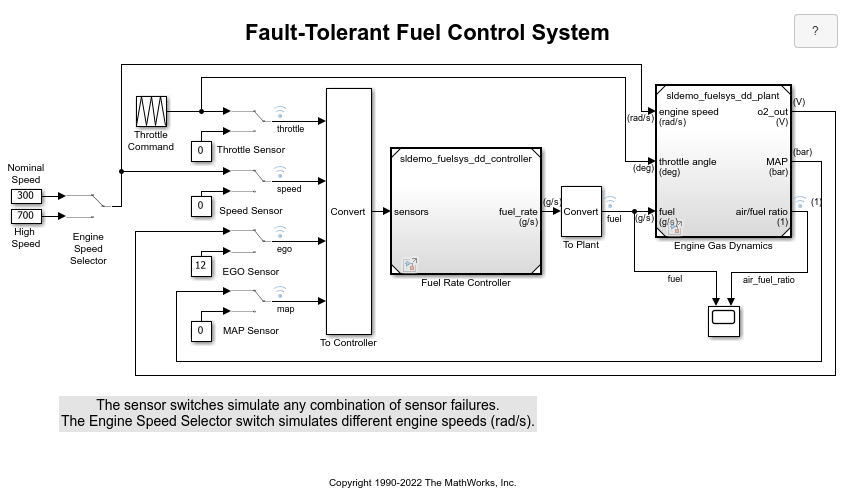# Switch Between Sets of Parameter Values During Simulation and Code Execution

To store multiple independent sets of values for the same block parameters, you can use an array of structures. To switch between the parameter sets, create a variable that acts as an index into the array, and change the value of the variable. You can change the value of the variable during simulation and, if the variable is tunable, during execution of the generated code.

### Explore Example Model

Open the example model.

```open_system('sldemo_fuelsys_dd_controller') ```This model represents the fueling system of a gasoline engine. The output of the model is the rate of fuel flow to the engine.

Navigate to the `switchable_compensation` nested subsystem.

```open_system(['sldemo_fuelsys_dd_controller/fuel_calc/',... 'switchable_compensation']) ```This subsystem corrects and filters noise out of the fuel rate signal. The subsystem uses different filter coefficients based on the fueling mode, which the control logic changes based on sensor failures in the engine. For example, the control algorithm activates the `low_mode` subsystem during normal operation. It activates the `rich_mode` subsystem in response to sensor failure.

Open the `low_mode` subsystem.

```open_system(['sldemo_fuelsys_dd_controller/fuel_calc/',... 'switchable_compensation/low_mode']) ```The Discrete Filter block filters the fuel rate signal. In the block dialog box, the Numerator parameter sets the numerator coefficients of the filter.

The sibling subsystem `rich_mode` also contains a Discrete Filter block, which uses different coefficients.

Update the model diagram to display the signal data types. The input and output signals of the block use the single-precision, floating-point data type `single`.

In the lower-left corner of the model, click the model data badge, then click the Data Dictionary link. The data dictionary for this model, `sldemo_fuelsys_dd_controller.sldd`, opens in the Model Explorer.

In the Model Explorer Model Hierarchy pane, select the Design Data node.

In the Contents pane, view the properties of the `Simulink.NumericType` objects, such as `s16En15`. All of these objects currently represent the single-precision, floating-point data type `single`. The model uses these objects to set signal data types, including the input and output signals of the Discrete Filter blocks.

Suppose that during simulation and execution of the generated code, you want each of these subsystems to switch between different numerator coefficients based on a variable whose value you control.

### Store Parameter Values in Array of Structures

Store the existing set of numerator coefficients in a `Simulink.Parameter` object whose value is a structure. Each field of the structure stores the coefficients for one of the Discrete Filter blocks.

```lowBlock = ['sldemo_fuelsys_dd_controller/fuel_calc/'... 'switchable_compensation/low_mode/Discrete Filter']; richBlock = ['sldemo_fuelsys_dd_controller/fuel_calc/'... 'switchable_compensation/rich_mode/Discrete Filter']; params.lowNumerator = eval(get_param(lowBlock,'Numerator')); params.richNumerator = eval(get_param(richBlock,'Numerator')); params = Simulink.Parameter(params); ```

Copy the value of `params` into a temporary variable. Modify the field values in this temporary structure, and assign the modified structure as the second element of `params`.

```temp = params.Value; temp.lowNumerator = params.Value.lowNumerator * 2; temp.richNumerator = params.Value.richNumerator * 2; params.Value(2) = temp; clear temp ```

The value of `params` is an array of two structures. Each structure stores one set of filter coefficients.

### Create Variable to Switch Between Parameter Sets

Create a `Simulink.Parameter` object named `Ctrl`.

```Ctrl = Simulink.Parameter(2); Ctrl.DataType = 'uint8'; ```

In the `low_mode` subsystem, in the Discrete Filter block dialog box, set the Numerator parameter to the expression `params(Ctrl).lowNumerator`.

```set_param(lowBlock,'Numerator','params(Ctrl).lowNumerator'); ```

In the Discrete Filter block in the `rich_mode` subsystem, set the value of the Numerator parameter to `params(Ctrl).richNumerator`.

```set_param(richBlock,'Numerator','params(Ctrl).richNumerator'); ```

The expressions select one of the structures in `params` by using the variable `Ctrl`. The expressions then dereference one of the fields in the structure. The field value sets the values of the numerator coefficients.

To switch between the sets of coefficients, you change the value of `Ctrl` to the corresponding index in the array of structures.

### Use Bus Object as Data Type of Array of Structures

Optionally, create a `Simulink.Bus` object to use as the data type of the array of structures. You can:

• Control the shape of the structures.

• For each field, control characteristics such as data type and physical units.

• Control the name of the `struct` type in the generated code.

Use the function `Simulink.Bus.createObject` to create the object and rename the object as `paramsType`.

```Simulink.Bus.createObject(params.Value) paramsType = slBus1; clear slBus1 ```

You can use the `Simulink.NumericType` objects from the data dictionary to control the data types of the structure fields. In the bus object, use the name of a data type object to set the `DataType` property of each element.

```paramsType.Elements(1).DataType = 's16En15'; paramsType.Elements(2).DataType = 's16En7'; ```

Use the bus object as the data type of the array of structures.

```params.DataType = 'Bus: paramsType'; ```

### Use Enumerated Type for Switching Variable

Optionally, use an enumerated type as the data type of the switching variable. You can associate each of the parameter sets with a meaningful name and restrict the allowed values of the switching variable.

Create an enumerated type named `FilterCoeffs`. Create an enumeration member for each of the structures in `params`. Set the underlying integer value of each enumeration member to the corresponding index in `params`.

```Simulink.defineIntEnumType('FilterCoeffs',{'Weak','Aggressive'},[1 2]) ```

Use the enumerated type as the data type of the switching variable. Set the value of the variable to `Aggressive`, which corresponds to the index `2`.

```Ctrl.Value = FilterCoeffs.Aggressive; ```

### Add New Objects to Data Dictionary

Add the objects that you created to the data dictionary `sldemo_fuelsys_dd_controller.sldd`.

```dictObj = Simulink.data.dictionary.open('sldemo_fuelsys_dd_controller.sldd'); sectObj = getSection(dictObj,'Design Data'); addEntry(sectObj,'Ctrl',Ctrl) addEntry(sectObj,'params',params) addEntry(sectObj,'paramsType',paramsType) ```

You can also store enumerated types in data dictionaries. However, you cannot import the enumerated type in this case because you cannot save changes to `sldemo_fuelsys_dd_controller.sldd`. For more information about storing enumerated types in data dictionaries, see Enumerations in Data Dictionary (Simulink).

### Switch Between Parameter Sets During Simulation

Open the example model `sldemo_fuelsys_dd`, which references the controller model `sldemo_fuelsys_dd_controller`.

```open_system('sldemo_fuelsys_dd') ```Set the simulation stop time to `Inf` so that you can interact with the model during simulation.

Begin a simulation run and open the Scope block dialog box. The scope shows that the fuel flow rate (the `fuel` signal) oscillates with significant amplitude during normal operation of the engine.

In the Model Explorer, view the contents of the data dictionary `sldemo_fuelsys_dd_controller.sldd`. Set the value of `Ctrl` to `FilterCoeffs.Weak`.

Update the `sldemo_fuelsys_dd` model diagram. The scope shows that the amplitude of the fuel rate oscillations decreases due to the less aggressive filter coefficients.

Stop the simulation.

### Generate and Inspect Code

If you have Simulink Coder software, you can generate code that enables you to switch between the parameter sets during code execution.

In the Model Explorer, view the contents of the data dictionary `sldemo_fuelsys_dd_controller.sldd`. In the Contents pane, set Column View to `Storage Class`.

Use the StorageClass column to apply the storage class `ExportedGlobal` to `params` so that the array of structures appears as a tunable global variable in the generated code. Apply the same storage class to `Ctrl` so that you can change the value of the switching variable during code execution.

Alternatively, to configure the objects, use these commands:

```tempEntryObj = getEntry(sectObj,'params'); params = getValue(tempEntryObj); params.StorageClass = 'ExportedGlobal'; setValue(tempEntryObj,params); tempEntryObj = getEntry(sectObj,'Ctrl'); Ctrl = getValue(tempEntryObj); Ctrl.StorageClass = 'ExportedGlobal'; setValue(tempEntryObj,Ctrl); ```

Generate code from the controller model.

```rtwbuild('sldemo_fuelsys_dd_controller') ```
```### Starting build procedure for: sldemo_fuelsys_dd_controller ### Successful completion of code generation for: sldemo_fuelsys_dd_controller ```

In the code generation report, view the header file `sldemo_fuelsys_dd_controller_types.h`. The code defines the enumerated data type `FilterCoeffs`.

```file = fullfile('sldemo_fuelsys_dd_controller_ert_rtw',... 'sldemo_fuelsys_dd_controller_types.h'); rtwdemodbtype(file,'#ifndef DEFINED_TYPEDEF_FOR_FilterCoeffs_',... '/* Forward declaration for rtModel */',1,0) ```
```#ifndef DEFINED_TYPEDEF_FOR_FilterCoeffs_ #define DEFINED_TYPEDEF_FOR_FilterCoeffs_ typedef enum { Weak = 1, /* Default value */ Aggressive } FilterCoeffs; #endif ```

The code also defines the structure type `paramsType`, which corresponds to the `Simulink.Bus` object. The fields use the single-precision, floating-point data type from the model.

```rtwdemodbtype(file,'#ifndef DEFINED_TYPEDEF_FOR_paramsType_',... '#ifndef DEFINED_TYPEDEF_FOR_FilterCoeffs_',1,0) ```
```#ifndef DEFINED_TYPEDEF_FOR_paramsType_ #define DEFINED_TYPEDEF_FOR_paramsType_ typedef struct { real32_T lowNumerator; real32_T richNumerator; } paramsType; #endif ```

View the source file `sldemo_fuelsys_dd_controller.c`. The code uses the enumerated type to define the switching variable `Ctrl`.

```file = fullfile('sldemo_fuelsys_dd_controller_ert_rtw',... 'sldemo_fuelsys_dd_controller.c'); rtwdemodbtype(file,'FilterCoeffs Ctrl = Aggressive;',... '/* Block signals (default storage) */',1,0) ```
```FilterCoeffs Ctrl = Aggressive; /* Variable: Ctrl * Referenced by: * '<S12>/Discrete Filter' * '<S13>/Discrete Filter' */ ```

The code also defines the array of structures `params`.

```rtwdemodbtype(file,'/* Exported block parameters */',... '/* Variable: params',1,1) ```
```/* Exported block parameters */ paramsType params = { { { 8.7696F, -8.5104F }, { 0.0F, 0.2592F } }, { { 17.5392F, -17.0208F }, { 0.0F, 0.5184F } } } ; /* Variable: params ```

The code algorithm in the model `step` function uses the switching variable to index into the array of structures.

To switch between the parameter sets stored in the array of structures, change the value of `Ctrl` during code execution.

Close the connections to the data dictionary. This example discards unsaved changes. To save the changes, use the `'-save'` option.

```Simulink.data.dictionary.closeAll('sldemo_fuelsys_dd_controller.sldd','-discard') ```